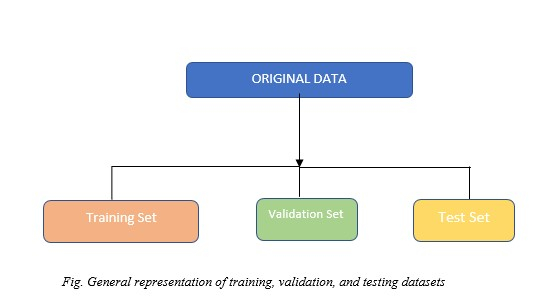# Training vs Testing vs Validation Sets

In this article, we are going to learn about the difference between – Training, Testing, and Validation sets

## Introduction

Data splitting is one of the simplest preprocessing techniques we can use in a Machine Learning/Deep Learning task. The original dataset is split into subsets like training, test, and validation sets. One of the prime reasons this is done is to tackle the problem of overfitting. However, there are other benefits as well. Let's have a brief understanding of these terms and see how they are useful.

## Training Set

The training set is used to fit or train the model. These data points are used to learn the parameters of the model. This is the biggest of all sets in terms of size. The training set includes the features and well as labels in the case of supervised learning. In the case of unsupervised learning, it can simply be the feature sets. These labels are used in the training phase to get the training accuracy score. The training set is usually taken as 70% of the original dataset but can be changed per the use case or available data.

For example

• While using Linear Regression, the points in the training set are used to draw the line of best fit.

• In K-Nearest Neighbors, the points in the training set are the points that could be the neighbors.### Applications of Train Set

Training sets are used in supervised learning procedures in data mining (i.e., classification of records or prediction of continuous target values.)

#### Example

Let’s consider a dataset containing 20 points
Dataset1 = [1,5,6,7,8,6,4,5,6,7,23,45,12,34,45,1,7,7,8,0]

Train set can be taken as 60 % of the original Dataset1
The train set will contain 12 data points [8,6,4,5,6,7,23,45,12,34,1,5]


## Validation Set

The validation set is used to provide an unbiased evaluation of the model fit during hyperparameter tuning of the model. It is the set of examples that are used to change learning process parameters. Optimal values of hyperparameters are tested against the model trained using the training set. In Machine Learning or Deep Learning, we generally need to test multiple models with different hyperparameters and check which model gives the best result. This process is carried out with the help of a validation set.

For example, in deep LSTM networks, a validation set is used to find the number of hidden layers, number of nodes, Dense units, etc.

### Applications of Validation Set

Validations sets are used for Hyperparameter tuning of AI models. Domains include Healthcare, Analytics, Cyber Security, etc.

#### Example

Let’s consider a dataset containing 20 points
Dataset2 = [1,5,6,7,8,6,4,5,6,7,23,45,12,34,45,1,7,7,8,0]

The validation set can be taken as 20 % of the original Dataset2.
The validation set will contain 4 data points [45,1,7,7]


## Testing Set

Once we have the model trained with the training set and the hyperparameter tuned using the validation set, we need to test whether the model can generalize well on unseen data. To accomplish this, a test set is used. Here we can check and compare the training and test accuracies. To ensure that the model is not overfitting or underfitting, test accuracies are highly useful. If there is a large difference in train and test accuracies, overfitting might have occurred.

While choosing the test set the below points should be kept in mind:

• The test should contain the same characteristics as of the train set.

• It should be large enough to yield statistically significant results

### Applications of Test Set

Test sets are used for evaluating metrics like:
Precision, Recall, AUC - ROC Curve, F1-Score


#### Example

Let's consider a data set containing 20 points
Dataset3 = [1,5,6,7,8,6,4,5,6,7,23,45,12,34,45,1,7,7,8,0]

The test set can be taken as 20 % of the original Dataset2
The test set will contain 4 data points [6,7,8,0]


### Why do we need a train, validation, and test sets?

The training set is necessary to train the model and learn the parameters. Almost all Machine learning/Deep Learning tasks should contain at least a training set.

The validation set and test sets are optional but highly recommended to use because only then can a trained model's legibility and accuracy can be verified. The validation set can be omitted if we do not choose to perform hyperparameter tuning or model selection. In such cases, a train set and test set will do the job.

A smart way to evaluate a model is to use K-Fold cross-validation.

The below table summarizes Training, Validation, and Testing sets.

Training Set Validation Set Testing Set
It is used to fit the model to learn the parameters of the model It is used to provide an unbiased evaluation of the model fit during hyperparameter tuning of the model It is used to test whether the model can generalize well on unseen data.
Larger in size as compared to validation and test sets Smaller in size. Smaller in size as compared to the train set.
In the case of supervised learning, it comprises features and labels. In unsupervised learning, it includes only features Contains both features and labels in supervised learning and only features in supervised learning Contains both features and labels in supervised learning and only features in supervised learning
Slower on larger datasets but the job can be run in parallel using multiprocessing Usually slower on a single core, if hyperparameters under observation are large. Can be run in parallel. Faster than both train and validation sets. Used to get the metrics on test data based on the trained model

## Conclusion

Splitting datasets for training, validation and testing is one of the backbone tasks for any Machine Learning or Deep Learning use case. It is highly simple, easily achievable, and resolves some of the very common problems like overfitting and underfitting.